# C/C++ program to make a simple calculator

• Difficulty Level : Easy
• Last Updated : 22 Nov, 2018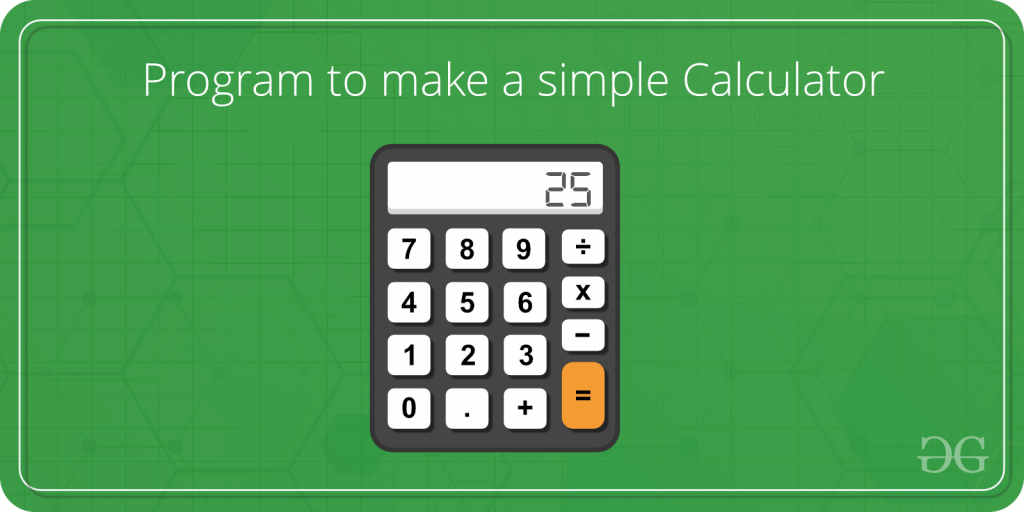Calculators are widely used device nowadays. It makes calculations easier and faster. Calculators are used to everyone in daily life. A simple calculator can be made using a C++ program which is able to add, subtract, multiply and divide, two operands entered by the user. The switch and break statement is used to create a calculator.

Program:

 `// C++ program to create calculator using``// switch statement``#include ``using` `namespace` `std;`` ` `// Main program``main()``{``    ``char` `op;``    ``float` `num1, num2;`` ` `    ``// It allows user to enter operator i.e. +, -, *, /``    ``cin >> op;`` ` `    ``// It allow user to enter the operands``    ``cin >> num1 >> num2;`` ` `    ``// Switch statement begins``    ``switch` `(op) {``         ` `        ``// If user enter + ``        ``case` `'+'``:``            ``cout << num1 + num2;``            ``break``;``         ` `        ``// If user enter - ``        ``case` `'-'``:``            ``cout << num1 - num2;``            ``break``;``         ` `        ``// If user enter *``        ``case` `'*'``:``            ``cout << num1 * num2;``            ``break``;``         ` `        ``// If user enter /``        ``case` `'/'``:``            ``cout << num1 / num2;``            ``break``;``         ` `        ``// If the operator is other than +, -, * or /, ``        ``// error message will display``        ``default``:``            ``cout << ``"Error! operator is not correct"``;``            ``break``;``    ``} ``// switch statement ends`` ` `    ``return` `0; ``}`

Output:

• Click on the run ide button, another tab will be open with web address
“https://ide.geeksforgeeks.org/index.php”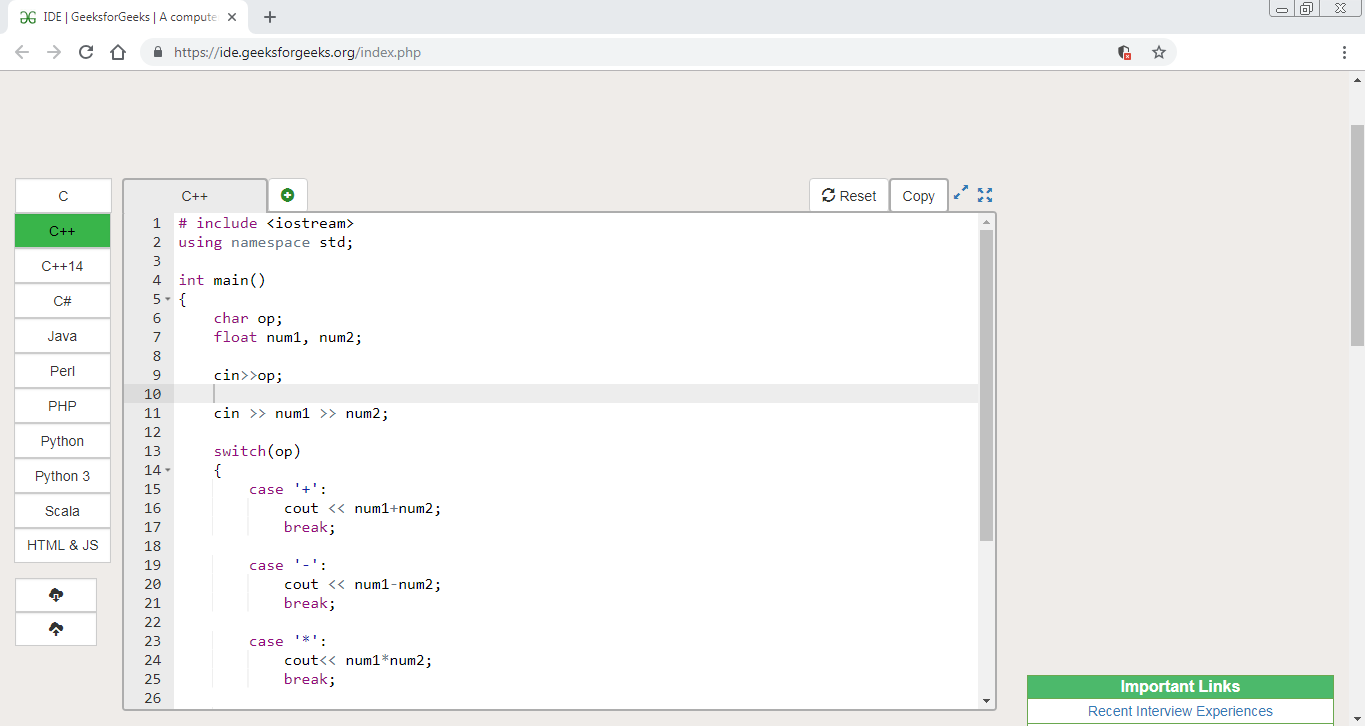• Put the input in the input box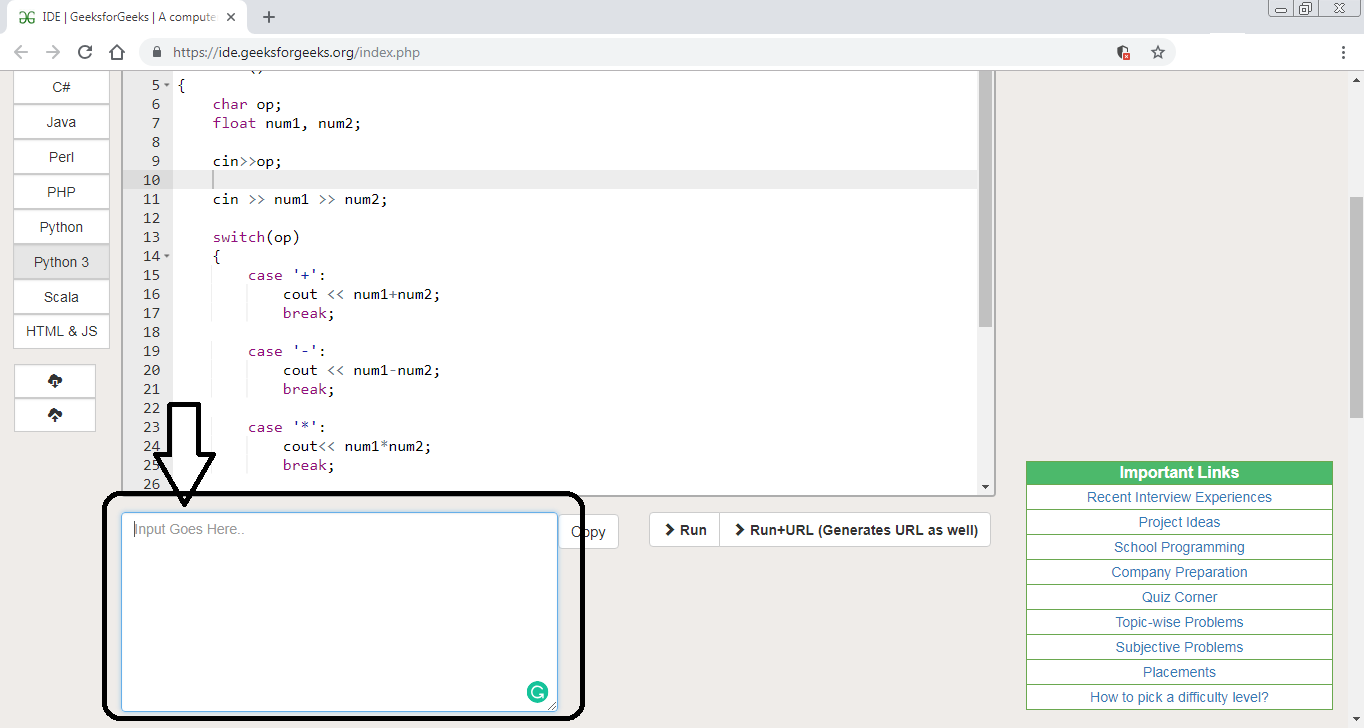• Enter the arithmetic operator (i.e.: either +, -, * or /) then, enter two operands on which need to perform the calculation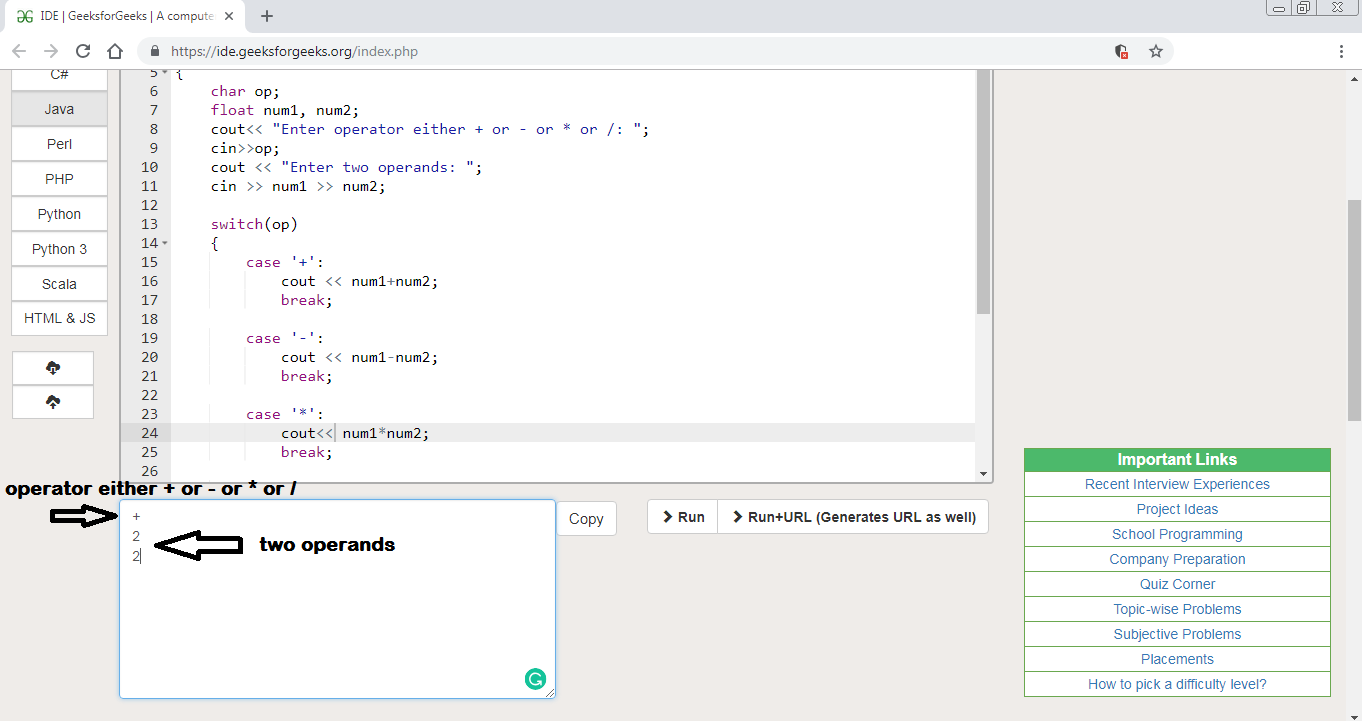• Click on the run button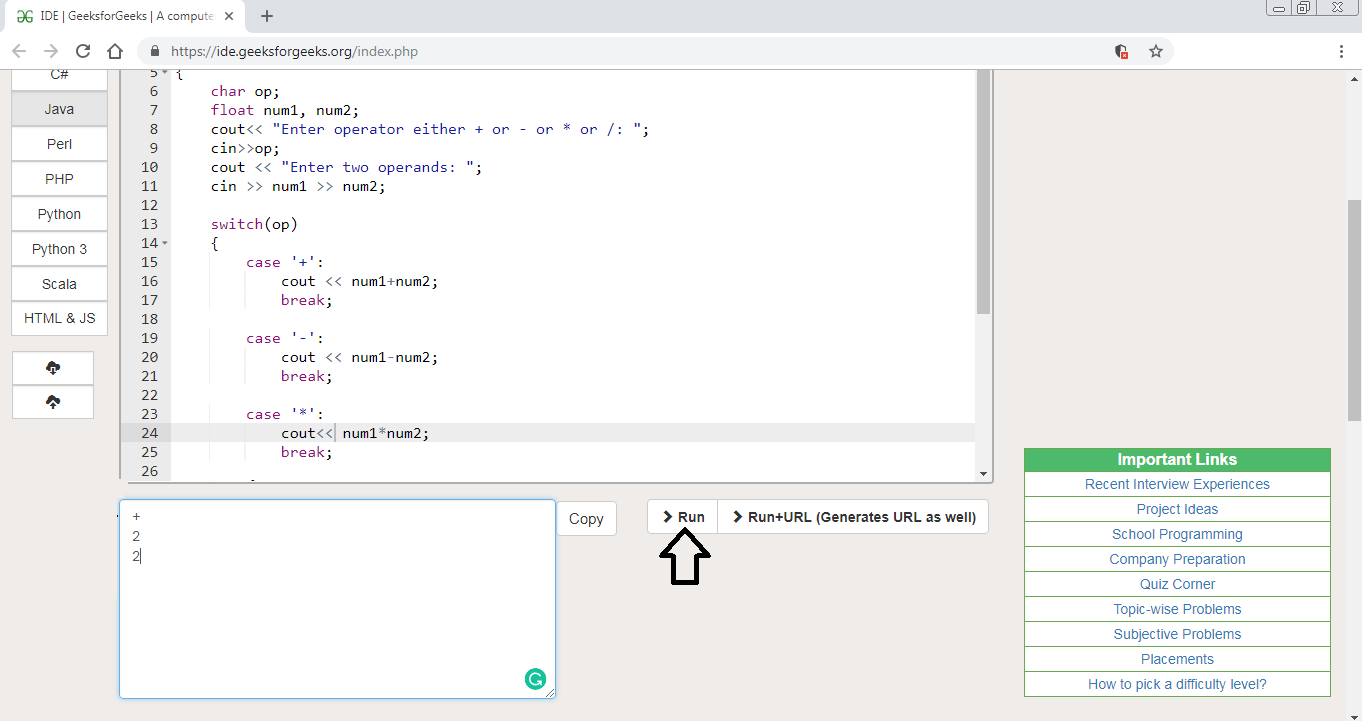• Then output box will appear with output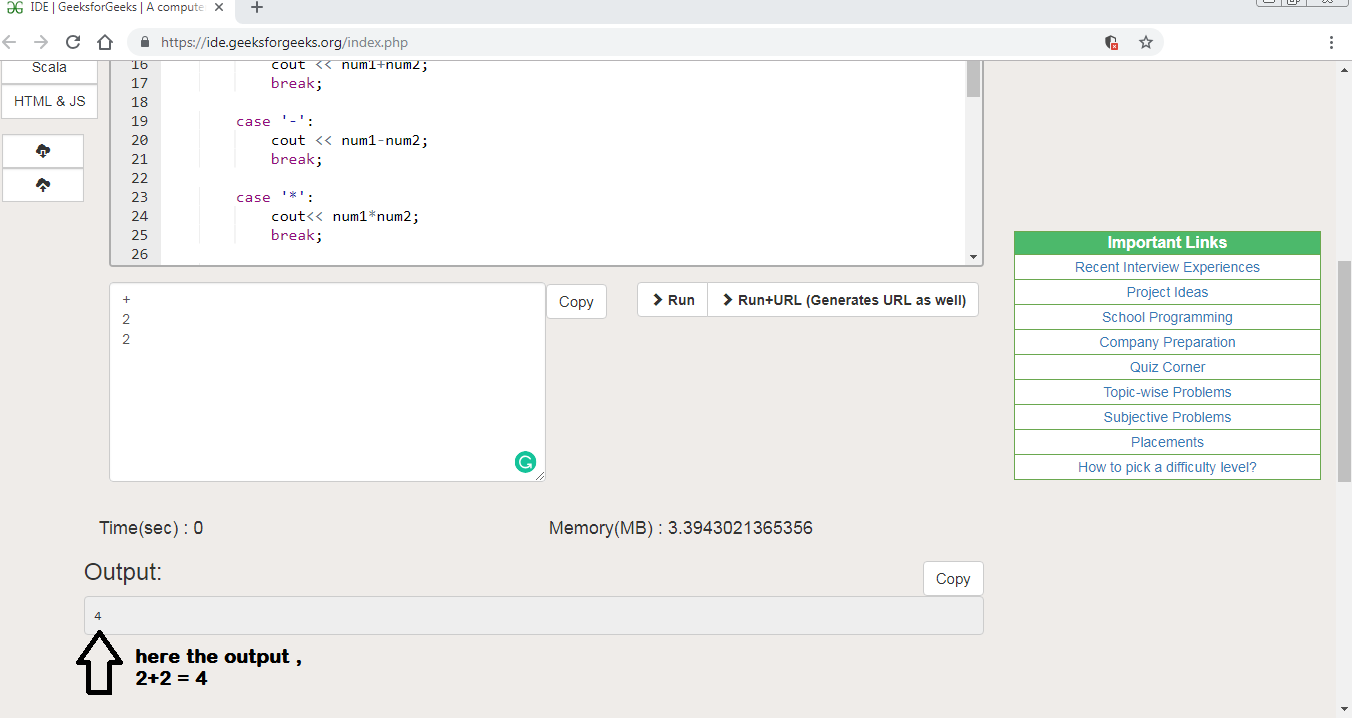My Personal Notes arrow_drop_up